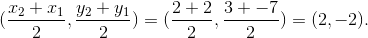# GMAT Math : Calculating the midpoint of a line segment

## Example Questions

### Example Question #1 : Coordinate Geometry

Which of the following quadrants can contain the midpoint of a line segment with endpoints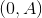and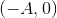for some nonzero value of?

Explanation:

The midpoint of the line segment with endpointsandis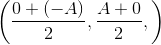, or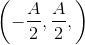If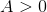, then the-coordinate is negative and the-coordinate is positive, so the midpoint is in Quadrant II. If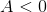, the reverse is true, so the midpoint is in Quadrant IV.

### Example Question #2 : Coordinate Geometry

The midpoint of a line segment with endpoints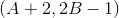and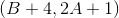is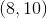. Sove for.It cannot be determined from the information given.It cannot be determined from the information given.

Explanation:

The midpoint of a line segment with endpoints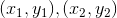is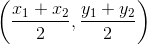.

Substitute the coordinates of the endpoints, then set each equation to the appropriate midpoint coordinate.-coordinate: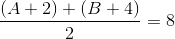-coordinate: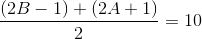Simplify each, then solve the system of linear equations in two variables: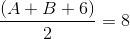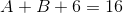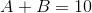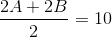The two linear equations turn out to be equivalent, meaning that there are infinitely many solutions to the system. Therefore, insufficient information is given to answer the question.

### Example Question #3 : Coordinate Geometry

Find the midpoint of the points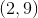and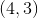.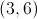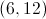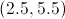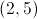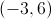Explanation:

Add the corresponding points together and divide both values by 2: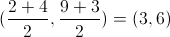### Example Question #4 : Coordinate Geometry

What is the midpoint of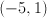and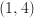?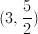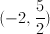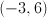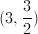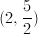Explanation:

Add the x-values and divide by 2, and then add the y-values and divide by 2.  Be careful of the negatives!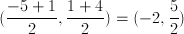### Example Question #5 : Coordinate Geometry

Consider segment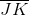which passes through the points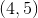and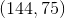.

What are the correct coordinates for the midpoint of?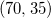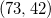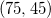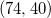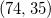Explanation:

Midpoint formula is as follows: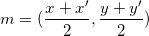Plug in and calculate: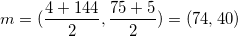### Example Question #6 : Coordinate Geometry

Segment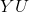has endpoints of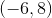and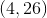. If the midpoint ofis given by point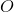, what are the coordinates of point?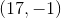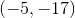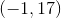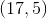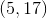Explanation:

Midpoints can be found using the following: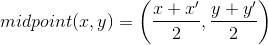Plug in our points (-6,8) and (4,26) to find the midpoint.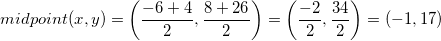### Example Question #7 : Coordinate Geometry

What are the coordinates of the mipdpoint of the line segment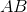if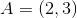and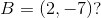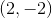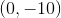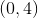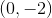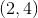The midpoint formula is